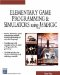` x = GetDegree(y) `

or

` y = GetRadians(x) `

### Parameters

 x The angle in degrees y The angle in radians

### Notes

Use GetDegree if you must, but you can do the conversion yourself very easily. Just remember that 2 pi radians corresponds to 360 degrees, so:

` Radians *57.3 = degrees Degrees/57.3 = radians `

Most program users will be unfamiliar or uncomfortable with radians, so if you are printing angles to the screen, degrees are the way to go.

On the other hand, most functions (like sine, cosine and tangent) in Jamagic require the angle to be in radians.

### Sample Code

` oworld = New World(); ocamera = New Camera(oworld); sphere = oworld.CreateSphere(50,50,50,25,25); ocamera.MoveBack(1200); sphere.Walk(); //Loop While(TRUE)  {   z = sphere.getyangle();   Y = sin(z);   // Convert to degrees.   X = z* 57.3   SetText("yangle " + x + "cos " + y);   } `Elementary Game Programming & Simulations Using Jamagic (Charles River Media Game Development)
ISBN: 1584502614
EAN: 2147483647
Year: 2002
Pages: 105
Authors: Sergio Perez